### ODS Graphics

Statistical procedures use ODS Graphics to create graphs as part of their output. ODS Graphics is described in detail in Chapter 21: Statistical Graphics Using ODS.

Before you create graphs, ODS Graphics must be enabled (for example, by specifying the ODS GRAPHICS ON statement). For more information about enabling and disabling ODS Graphics, see the section Enabling and Disabling ODS Graphics in Chapter 21: Statistical Graphics Using ODS.

The overall appearance of graphs is controlled by ODS styles. Styles and other aspects of using ODS Graphics are discussed in the section A Primer on ODS Statistical Graphics in Chapter 21: Statistical Graphics Using ODS.

These ODS graphs are controlled by the PLOTS= option in the PROC PROBIT statement. You can specify more than one graph request with the PLOTS= option. Table 75.41 summarizes these requests.

Table 75.41: Options for Plots

Option

Plot

ALL

All appropriate plots

CDFPLOT

Estimated cumulative probability

IPPPLOT

Inverse predicted probability

LPREDPLOT

Linear predictor

NONE

No plot

PREDPPLOT

Predicted probability

The following subsections provide information about these graphs.

#### ODS Graph Names

PROC PROBIT assigns a name to each graph it creates using ODS. You can use these names to reference the graphs when using ODS. The names are listed in Table 75.42.

Table 75.42: Graphs Produced by PROC PROBIT

ODS Graph Name

Plot Description

Statement

PLOTS= Option

CDFPlot

Estimated cumulative probability

PROC

CDFPLOT

IPPPlot

Inverse predicted probability

PROC

IPPPLOT

LPredPlot

Linear predictor

PROC

LPREDPLOT

PredPPlot

Predicted probability

PROC

PREDPPLOT

#### CDF Plot

For a multinomial model, the predicted cumulative distribution function is defined as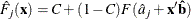where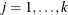are the indexes of the k levels of the multinomial response variable, F is the CDF of the distribution used to model the cumulative probabilities,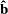is the vector of estimated parameters,is the covariate vector,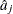are estimated ordinal intercepts with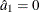, and C is the threshold parameter, either known or estimated from the model. Let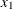be the covariate corresponding to the dose variable and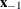be the vector of the rest of the covariates. Let the corresponding estimated parameters be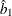and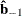. Then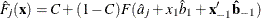To plot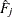as a function of,must be specified. You can use the XDATA= option to provide the values of(see the XDATA= option in the PROC PROBIT statement for details), or use the default values that follow these rules:

• If the effect contains a continuous variable (or variables), the overall mean of this effect is used.

• If the effect is a single classification variable, the highest level of the variable is used.

The LEVEL= suboption specify the levels of the multinomial response variable for which the CDF curves are requested. There are k – 1 curves for a k-level multinomial response variable (for the highest level, it is the constant line 1). You can specify any of them to be plotted by the LEVEL= suboption. See the plot in Output 75.2.6 for an example.

#### Inverse Predicted Probability Plot

For the binomial model, the response variable is a probability. An estimate of the dose level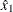needed for a response of p is given by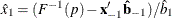where F is the cumulative distribution function used to model the probability,is the vector of the rest of the covariates,is the vector of the estimated parameters corresponding to, andis the estimated parameter for the dose variable of interest.

To plotas a function of p,must be specified. You can use the XDATA= option to provide the values of(see the XDATA= option in the PROC PROBIT statement for details), or use the default values that follow these rules:

• If the effect contains a continuous variable (or variables), the overall mean of this effect is used.

• If the effect is a single classification variable, the highest level of the variable is used.

Output 75.4.12 in Example 75.4 shows an inverse predicted probability plot.

#### Linear Predictor Plot

For both binomial models and multinomial models, the linear predictor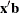can be plotted against the first single continuous variable (dose variable) in the MODEL statement.

Letbe the covariate of the dose variable,be the vector of the rest of the covariates,be the vector of estimated parameters corresponding to, andbe the estimated parameter for the dose variable of interest.

To plot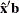as a function of,must be specified. You can use the XDATA= option to provide the values of(see the XDATA= option in the PROC PROBIT statement for details), or use the default values that follow these rules:

• If the effect contains a continuous variable (or variables), the overall mean of this effect is used.

• If the effect is a single classification variable, the highest level of the variable is used.

For the multinomial model, you can use the LEVEL= suboption to specify the levels for which the linear predictor lines are plotted.

The confidence limits for the predicted values are only available for the binomial model. Output 75.4.13 in Example 75.4 shows a linear predictor plot for a binomial model.

#### Predicted Probability Plot

The predicted probability is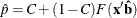for the binomial model and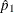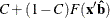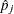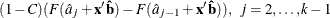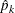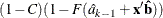for the multinomial model with k response levels, where F is the cumulative distribution function used to model the probability,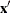is the vector of the covariates,are the estimated ordinal intercepts with, C is the threshold parameter, either known or estimated from the model, and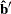is the vector of estimated parameters.

To plot
(or) as a function of a continuous variable, the remaining covariatesmust be specified. You can use the XDATA= option to provide the values of(see the XDATA= option in the PROC PROBIT statement for details), or use the default values that follow these rules:

• If the effect contains a continuous variable (or variables), the overall mean of this effect is used.

• If the effect is a single classification variable, the highest level of the variable is used.

For the multinomial model, you can use the LEVEL= suboption to specify the levels for which the linear predictor lines are plotted.

Confidence limits are plotted only for the binomial model. Output 75.1.7 in Example 75.1 shows a predicted probability plot for a binomial model; and Output 75.2.3 in Example 75.2 shows a predicted probability plot for a multinomial model.